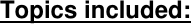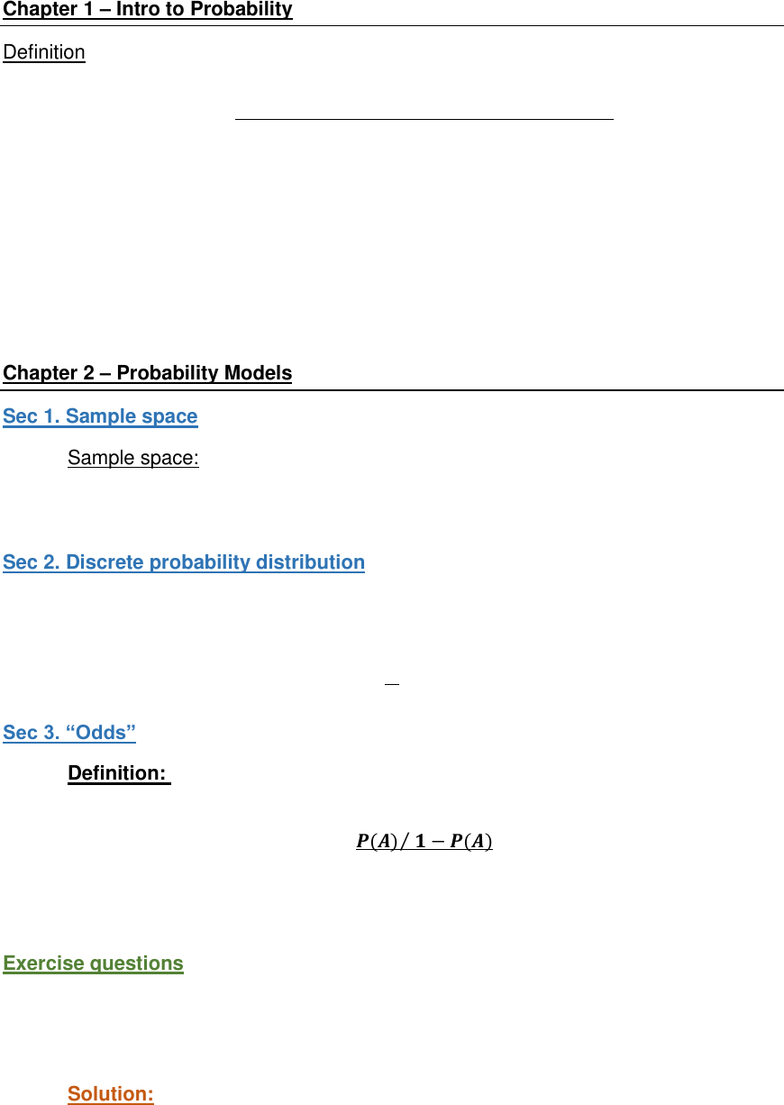# STAT230 Study Guide - Final Guide: Random Variable, Probability Distribution, Continuity Correction

191 views14 pagesUniversity of Waterloo
STAT 230
Probability
Fall 2018
Final Exam
Prof: Adam Kolkiewicz.
Exam Guide
Topics included:
1. Intro to probability
2. Probability Models
3. Counting techniques
4. Probability Rules and Conditional Probability
5. Discrete Random Variables
6. Expected Value and Variance
7. Continuous Random Variables
8. Multivariate Distributions
Unlock document

This preview shows pages 1-3 of the document.
Unlock all 14 pages and 3 million more documents.

Already have an account? Log inChapter 1 Intro to Probability
Definition
1. Classical definition: probability of some event is


2. Relative frequency:
- (limiting) proportion of times the event occurs in a very long series of
repetitions in the experiment
3. Subject probability definition
- A measure of how sure the person states something will happen.
Chapter 2 Probability Models
Sec 1. Sample space
1. Sample space: the set of all possible(distinct) outcome of the random experiment
2. Set of all subsets of the sample space: “events” going to assign probabilities
3. Mechanism for probabilities: [0, 1].
Sec 2. Discrete probability distribution
1. For a discrete sample space containing ai, 0P(ai) 1. And

2. Uniform Probability Model: for the sample space S with equally likely simple
events, we have the probability of every individual outcome is the same.

Sec 3. “Odds
Definition: The odds in favour of an event A is the probability with which the
event occurs divided by the probability it does not occur: assuming P(A) > 0.
The odds against the event is the reciprocal of the odds in favour.
Exercise questions
Question 1. Roll a six sided die.
a. What is the probability that the number is 6?
b. What is the probability that the number is less than 6?
c. What is the probability that the number is even?
Solution:
A = the rolled number is 6
B = the rolled number is less than 6
Unlock document

This preview shows pages 1-3 of the document.
Unlock all 14 pages and 3 million more documents.

Already have an account? Log inC = the rolled number is even
S = {1, 2, 3, 4, 5, 6}
a. Probability =  
=  
b. Probability =  
=  
c. Probability =  
=  
Question 2. Draw a card hand at random from a standard 52 card deck of
playing cards (13 kinds: A, 2, 3, 4,... 10, J, Q, K, in 4 suits).
i) What is the probability that the hand contains is diamond?
ii) What is the probability that the hand is smaller than 10?
Solution:
The events of interest:
A = the hand contains a diamond
B = the hand contains < 10
i) Probability =  
=  
= 1/4
ii) Probability =  
= 
= 9/13
Question 3. Draw a card hand at random from a standard 52 card deck of
playing cards (13 kinds: A, 2, 3, 4,... 10, J, Q, K, in 4 suits).
What is the odd in favour of choosing the diamond? And odd against?
Solution:
- If the probability that the selected card is diamond is ¼, then the odds in
favour of diamonds are ¼ / ¾ = 1/3. That is 1:3.
- So the odds against diamonds are 3:1.
Unlock document

This preview shows pages 1-3 of the document.
Unlock all 14 pages and 3 million more documents.

Already have an account? Log in

# Get OneClass Grade+

Unlimited access to all notes and study guides.

###### Grade+All Inclusive
\$10 USD/m
You will be charged \$120 USD upfront and auto renewed at the end of each cycle. You may cancel anytime under Payment Settings. For more information, see our Terms and Privacy.
Payments are encrypted using 256-bit SSL. Powered by Stripe.